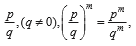# User Forum

Subject :IMO    Class : Class 7

Which of the following statements is INCORRECT?

AAny number raised to the power of one, equals the number itself.
BThe exponent is the number of times the base multiply itself.
CExponential notation is a concise way of denoting a number or an expression, that is repeatedly multiplied by itself.
DFor any rational numberwhere m is only a positive integer.

ALL OPTIONS ARE CORRECT